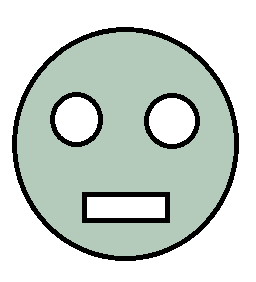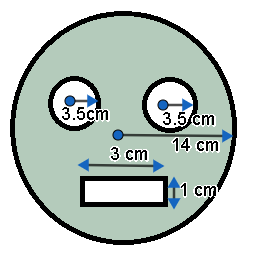Courses
Courses for Kids
Free study material
Offline Centres
MoreLast updated date: 02nd Dec 2023
Total views: 281.1k
Views today: 5.81k

# From a circular card sheet of radius 14cm, two circles of radius 3.5cm and a rectangle of length 3 cm and breadth 1 cm are removed (as shown in the adjoining figure). Find the area of the remaining sheet.(Take $\pi =\dfrac{22}{7}$ ).Verified
281.1k+ views
Hint: We have to find the area of the circular sheet, area of the two small circles and the area of the rectangle. We will be using the formulas $A=\pi {{r}^{2}}$ and $A=l\times b$ to find the area of the circle and rectangle respectively. To find the area of the remaining sheet, we have to subtract the sum of areas of the two small circles and the rectangle from the area of the circular sheet.

We are given the radius of the circular sheet is 14 cm.Let us find the area of the circular sheet. We know that area of a circle is given by
$A=\pi {{r}^{2}}$
where r is the radius of the circle.
Let us assume the area of the circular sheet as ${{A}_{1}}$ . We have to substitute the values in the above formula.
\begin{align} & \Rightarrow {{A}_{1}}=\pi \times {{14}^{2}} \\ & \Rightarrow {{A}_{1}}=14\times 14\times \dfrac{22}{7} \\ \end{align}
Let us cancel the common factors.
\begin{align} & \Rightarrow {{A}_{1}}={{\require{cancel}\cancel{14}}^{2}}\times 14\times \dfrac{22}{\require{cancel}\cancel{7}} \\ & \Rightarrow {{A}_{1}}=2\times 14\times 22 \\ & \Rightarrow {{A}_{1}}=616\text{ }c{{m}^{2}} \\ \end{align}
We are given that two circles of radius 3.5cm are removed. Let us find the area of one small circle.
$\Rightarrow {{A}_{2}}=\pi {{\left( 3.5 \right)}^{2}}$
We can write 3.5 as $\dfrac{35}{10}$ .
\begin{align} & \Rightarrow {{A}_{2}}=\dfrac{22}{7}\times {{\left( \dfrac{35}{10} \right)}^{2}} \\ & \Rightarrow {{A}_{2}}=\dfrac{22}{7}\times \dfrac{35}{10}\times \dfrac{35}{10} \\ \end{align}
Let us cancel the common factors.
\begin{align} & \Rightarrow {{A}_{2}}=\dfrac{{{\require{cancel}\cancel{22}}^{11}}}{\require{cancel}\cancel{7}}\times \dfrac{{{\require{cancel}\cancel{35}}^{\require{cancel}\cancel{5}}}}{{{\require{cancel}\cancel{10}}^{\require{cancel}\cancel{2}}}}\times \dfrac{{{\require{cancel}\cancel{35}}^{7}}}{{{\require{cancel}\cancel{10}}^{2}}} \\ & \Rightarrow {{A}_{2}}=11\times \dfrac{7}{2} \\ & \Rightarrow {{A}_{2}}=\dfrac{77}{2} \\ \end{align}
$\Rightarrow {{A}_{2}}=38.5\text{ }c{{m}^{2}}$
We are also given that a rectangle of length 3 cm and breadth 1 cm is also removed. Let us find the area of the rectangle. We know that area of a rectangle is given by
$A=l\times b$
where l is the length and b is the breadth of the rectangle.
Therefore, area of the given rectangle is
\begin{align} & \Rightarrow {{A}_{3}}=3\times 1 \\ & \Rightarrow {{A}_{3}}=3\text{ }c{{m}^{2}} \\ \end{align}
We have to find the area of the remaining sheet. We can obtain this area by subtracting the sum of areas of the two small circles and the rectangle from the area of the circular sheet.
$\text{Area of the remaining sheet}=\text{ Area of circular sheet}-\left( \text{Area of two small circle}+\text{Area of rectangle} \right)$We can write the areas as
$\Rightarrow \text{Area of the remaining sheet}={{A}_{1}}-\left( {{A}_{2}}+{{A}_{2}}+{{A}_{3}} \right)$
Let us substitute the values.
\begin{align} & \Rightarrow \text{Area of the remaining sheet}=616\text{ }-\left( 38.5+38.5+3 \right) \\ & \Rightarrow \text{Area of the remaining sheet}=616\text{ }-\left( 77+3 \right) \\ & \Rightarrow \text{Area of the remaining sheet}=616\text{ }-80 \\ & \Rightarrow \text{Area of the remaining sheet}=536\text{ c}{{\text{m}}^{2}} \\ \end{align}
Therefore, the area of the remaining sheet is 536 $\text{c}{{\text{m}}^{2}}$ .

Note: Students must be thorough with the areas of shapes like circles, squares, rectangles and triangles. They have a chance of making a mistake by writing the area of the circle as $2\pi r$ or the area of the rectangle as $2\left( l+b \right)$ . These are the perimeters of circle and rectangle respectively. Students have a chance of making a mistake by finding the remaining area of the sheet by subtracting the difference of areas of the two small circles and the rectangle from the area of the circular sheet.